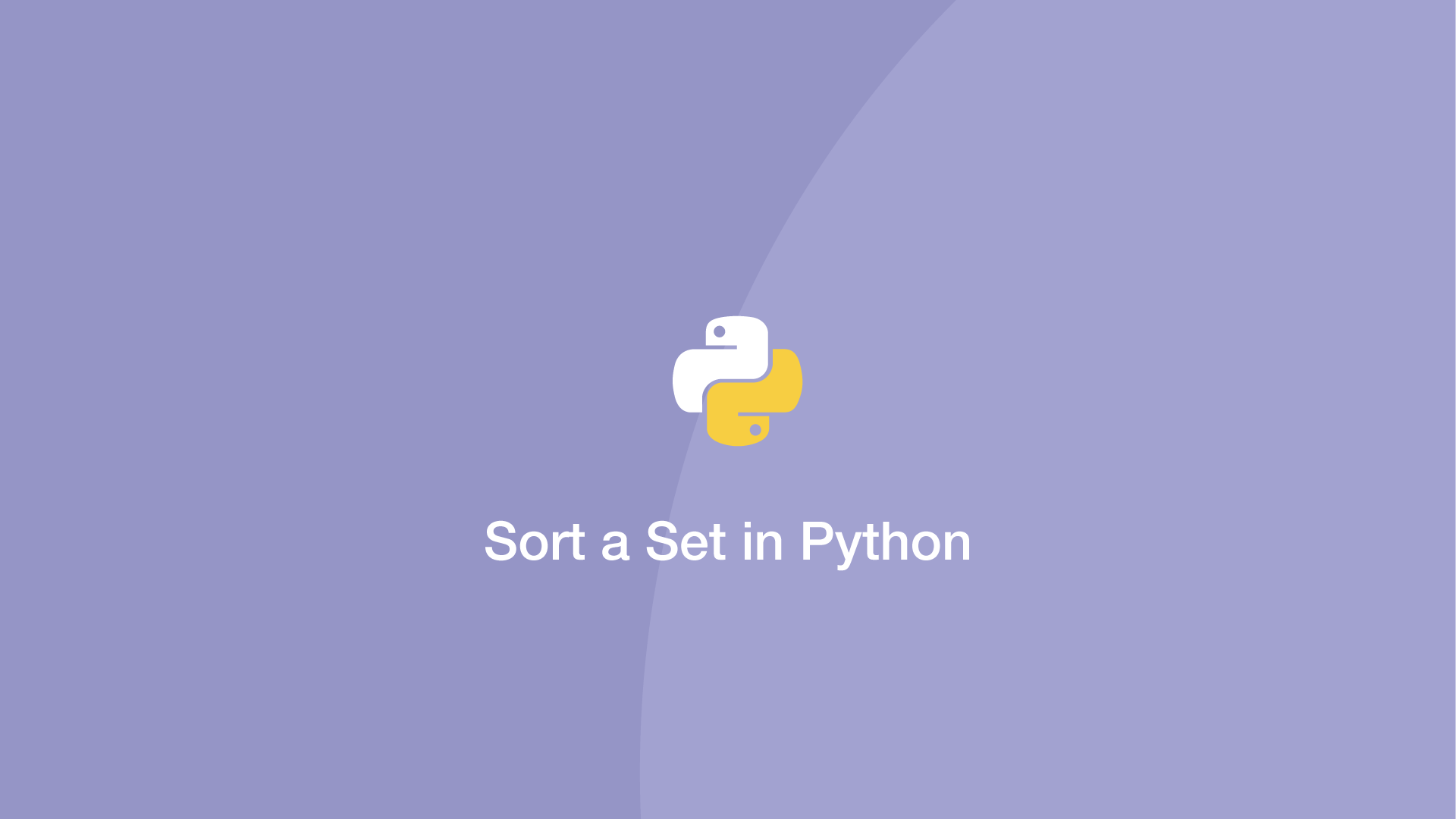# Sort a Set in PythonTo sort a Python set, use either the `sorted()` or `sort()` function. In this tutorial, we will go through examples of how to use both of these functions.

## How to use the Python sorted() function

Here is the syntax of the Python `sorted()` function:

``````sorted(iterable, key=key, reverse=reverse)
``````

• `iterable` – The iterable to sort (required).
• `key` – a function to run that changes the behaviour of the sorting (optional).
• `reverse` – boolean value to control sorting direction, True is reverse. Default is False (optional).

## Python Sorted Set Example

The example below demonstrates sorting a set of numbers into ascending order:

``````s = {11,5,7,1,9}
print(sorted(s))
``````
``````[1, 5, 7, 9, 11]
``````

`sorted()` returns a list, to convert it back to a set use the `set()` function like this:

``````s = {11,5,7,1,9}
print(set(sorted(s)))
``````
``````{1, 5, 7, 9, 11}
``````

## Sort a Set in Descending Order Example

Here is another example, this time sorting a set into descending order using the reverse attribute in the `sorted()` function:

``````s = {11,5,7,1,9}
res = sorted(s, reverse=True)
print(res)
``````
``````[11, 9, 7, 5, 1]
``````

Note - If you convert the result back to a set after completing the descending order operation it will automatically revert back to ascending order.

## Sort a Set with the Python set() Function

The `sort()` function only works with lists. To get around this, we can wrap the set() in list tags before sorting. The sort() function is destructive as it modifies the original list.

``````s = {11,5,7,1,9}
[s].sort()
print(s)
``````
``````{1, 5, 7, 9, 11}
``````

The advantage of using `sort()` is the result doesn't need to be converted back to a set:

``````s = {11,5,7,1,9}
[s].sort()
print(type(s))
``````
``````<class 'set'>
``````

#### Related Tutorials### How to Create and Use Lists (Arrays) in Python

September 01, 2020### How to Sort Dictionaries by Value In Python

September 22, 2020### Pretty Print a Dictionary in Python

September 16, 2021### How to Sort a list Alphabetically in Python

April 28, 2021### How to use Sets in Python

September 15, 2020### How to Parse JSON Data in Python (Read and Write)

September 18, 2020